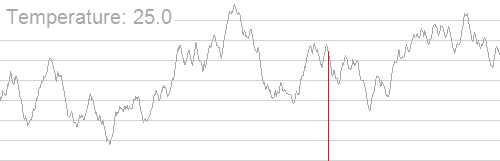# Problem

We need to optimize the global optimum of a given function on some space using only the values of the function in some points on the space.

Simulated Annealing is a probabilistic technique for approximating the global optimum of a given function.

# Algorithm

The name and inspiration come from annealing in metallurgy, a technique involving heating and controlled cooling of a material to increase the size of its crystals and reduce their defects. Both are attributes of the material that depend on its thermodynamic free energy. Heating and cooling the material affects both the temperature and the thermodynamic free energy. The simulation of annealing can be used to find an approximation of a global minimum for a function with many variables.

## Steps of the Algorithm

Step 1 Let $k = 0$ - current iteration, $T = T_k$ - initial temperature.

Step 2 Let $x_k \in X$ - some random point from our space

Step 3 Let decrease the temperature by following rule $T_{k+1} = \alpha T_k$ where $% $ - some constant that often is closer to 1

Step 4 Let $x_{k+1} = g(x_k)$ - the next point which was obtained from previous one by some random rule. It is usually assumed that this rule works so that each subsequent approximation should not differ very much.

Step 5 Calculate $\Delta E = E(x_{k+1}) - E(x_{k})$, where $E(x)$ - the function that determines the energy of the system at this point. It is supposed that energy has the minimum in desired value $x^*$.

Step 6 If $% $ then the approximation found is better than it was. So accept $x_{k+1}$ as new started point at the next step and go to the step Step 3

Step 7 If $\Delta E >= 0$, then we accept $x_{k+1}$ with the probability of $P(\Delta E) = \exp^{-\Delta E / T_k}$. If we don’t accept $x_{k+1}$, then we let $k = k+ 1$. Go to the step Step 3

The algorithm can stop working according to various criteria, for example, achieving an optimal state or lowering the temperature below a predetermined level $T_{min}$.

## Convergence

As it mentioned in Simulated annealing: a proof of convergence the algorithm converges almost surely to a global maximum.

## Illustration

A gif from Wikipedia:# Example

In our example we solve the N queens puzzle - the problem of placing N chess queens on an N×N chessboard so that no two queens threaten each other.## The Problem

Let $E(x)$ - the number of intersections, where $x$ - the array of placement queens at the field (the number in array means the column, the index of the number means the row).

The problem is to find $x^*$ where $E(x^*) = \min_{x \in X} E(x)$ - the global minimum, that is predefined and equals to 0 (no two queens threaten each other).

In this code $x_0 = [0,1,2,...,N]$ that means all queens are placed at the board’s diagonal . So at the begining $E = N(N-1)$, because every queen intersects others.

## Results

Results of applying this algorithm with $\alpha = 0.95$ to the $N$ queens puzzle for $N = 10$ averaged by 100 runs are below:Results of running the code for $N$ from $4$ to $40$ and measuring the time it takes to find the solution averaged by 100 runs are below: